Courses

# Test: Name Reaction- 1

## 30 Questions MCQ Test Organic Chemistry | Test: Name Reaction- 1

Description
This mock test of Test: Name Reaction- 1 for Chemistry helps you for every Chemistry entrance exam. This contains 30 Multiple Choice Questions for Chemistry Test: Name Reaction- 1 (mcq) to study with solutions a complete question bank. The solved questions answers in this Test: Name Reaction- 1 quiz give you a good mix of easy questions and tough questions. Chemistry students definitely take this Test: Name Reaction- 1 exercise for a better result in the exam. You can find other Test: Name Reaction- 1 extra questions, long questions & short questions for Chemistry on EduRev as well by searching above.
QUESTION: 1

Solution:
QUESTION: 2

Solution:
QUESTION: 3

### The major product formed in the following reaction is: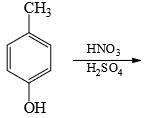Solution:
QUESTION: 4

Choose the reaction sequence that could be used to perform the following transformation: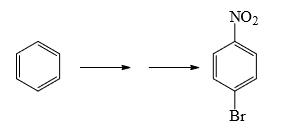Solution:
QUESTION: 5

What could be reagents A and B for the following reactions: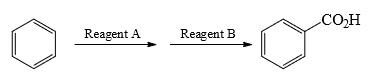Solution:
QUESTION: 6

What could be the reagent to complete the following reaction: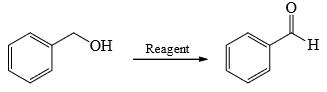Solution:
QUESTION: 7

Choose order that has the following compounds correctly arranged with respect to increasing rate of reaction with LiAlH4 (most reactive compound on the right).

Solution:
QUESTION: 8

Choose the reaction(s) that will not proceed as shown hereunder:

Solution:

Reduction of amide by LAH gives amine but hydrolysis of amine not give alcohol.

QUESTION: 9

Predict the product of the following aldol condensation: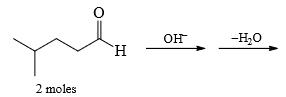Solution:
QUESTION: 10

Which of the following structure is more stable:

Solution:
QUESTION: 11

Which of the following is most reactive towards aqueous HBr:

Solution:
QUESTION: 12

Ethylbenzene when treated with chlorine in presence of light mainly gives:

Solution:
QUESTION: 13

The following alcohol is treated with Conc. H2SO4, the major product obtained is: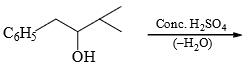Solution:
QUESTION: 14

The major product formed in the following reaction is: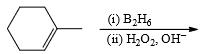Solution:
QUESTION: 15

Give the nature of And B in the given reaction: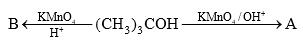Solution:
QUESTION: 16

The reactionis an example of:

Solution:
QUESTION: 17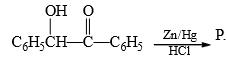Here, P should be:

Solution:
QUESTION: 18

Nitrobenzene can be reduced to aniline by:

(I) H2/Ni

(II) Sn/HCl

(III) Zn/NaOH

(IV) LiAlH4

Solution:

Explanation : When nitrobenzene is reduced with a metal and acid (Sn/HCl, Zn/HCl etc.), aniline is obtained. The intermediate products nitrosobenzene and phenylhydroxylamine are never isolated because these are reduced more rapidly than nitrobenzene.

However, the catalytic hydrogenation of nitrobenzene gives aniline.

PhNO2  (H2+Ni)------------->PhNH2

​

Azobenzene is formed when nitrobenzene is reduced by LiAlH4.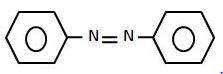QUESTION: 19

1-Methylcyclopentene can be converted into 2-methylcyclopentanol by:

Solution:
QUESTION: 20

2-Methylpropanol-2 can be obtained by the acid-catalyzed hydration of:

Solution:
QUESTION: 21

A new carbon-carbon bond formation is possible in

Solution:
QUESTION: 22

The major product formed in the following reaction is: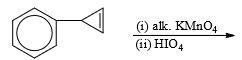Solution:
QUESTION: 23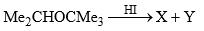Predict the nature of product and the type of reaction involved in their formation.

Solution:
QUESTION: 24

Anisole is treated with HI under two different conditions: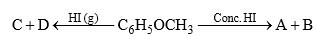The nature of A and D will be

Solution:
QUESTION: 25

The ethereal linkage (—C—O—C—) is cleaved by:

Solution:
QUESTION: 26

Predict the compounds A and B in the following reactions: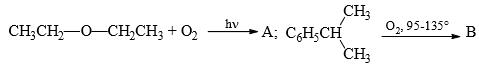Solution:
QUESTION: 27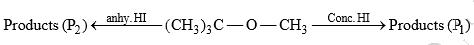The products P1 and P2 respectively are:

Solution: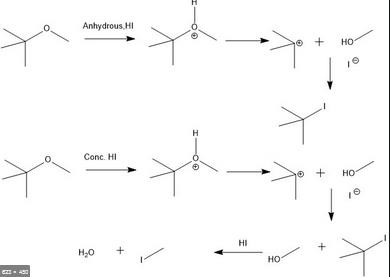QUESTION: 28

Which of the following statement is true regarding amount of AlCl3 required during Friedel-Craft acetylation using acetyl chloride or acetic anhydride:

Solution:
QUESTION: 29

Which of the following gives effervescences of CO2 with NaHCO3 solution:

Solution:
QUESTION: 30

2,4,6-Trinitrophenol can be prepared in good yield:

Solution: